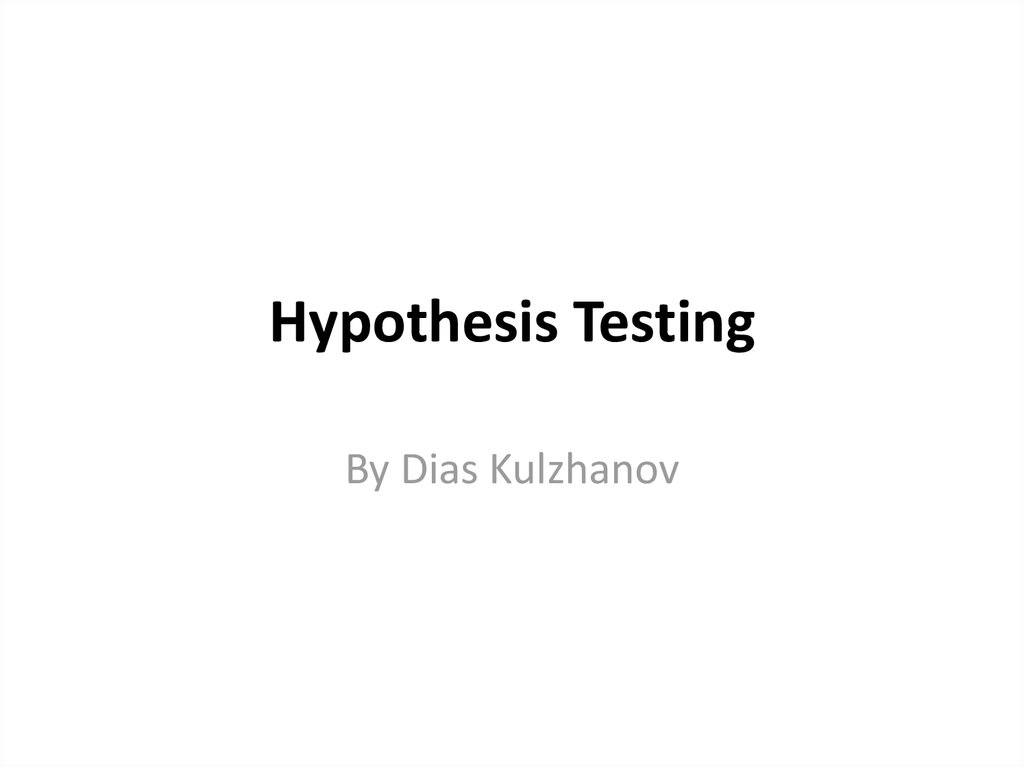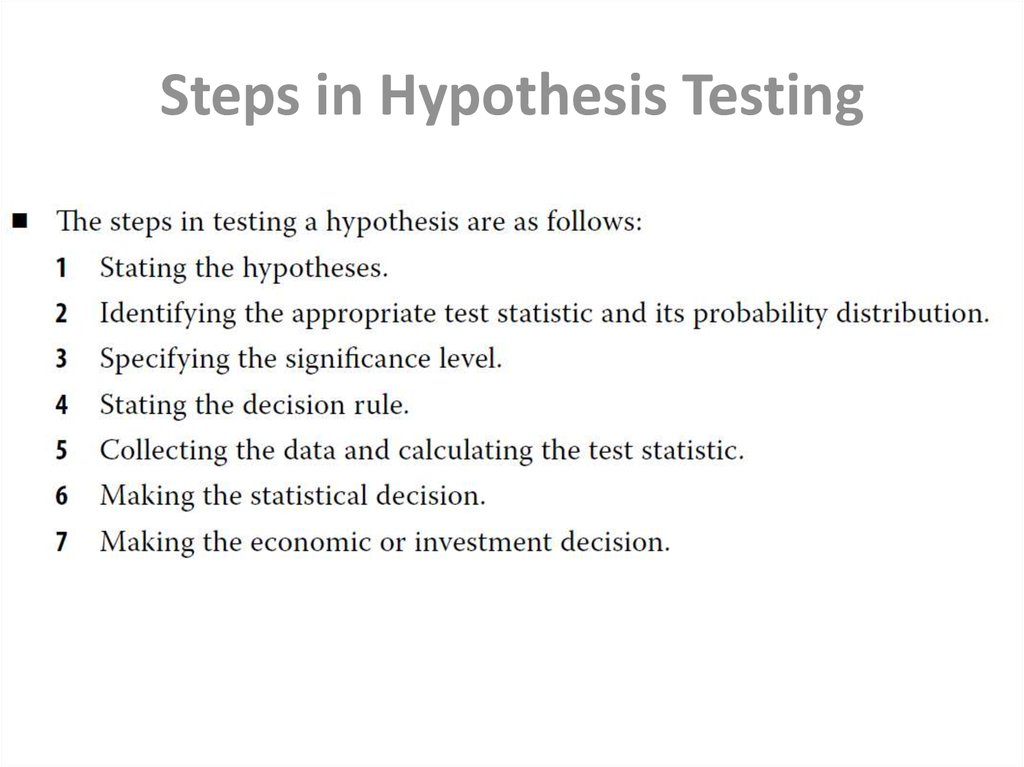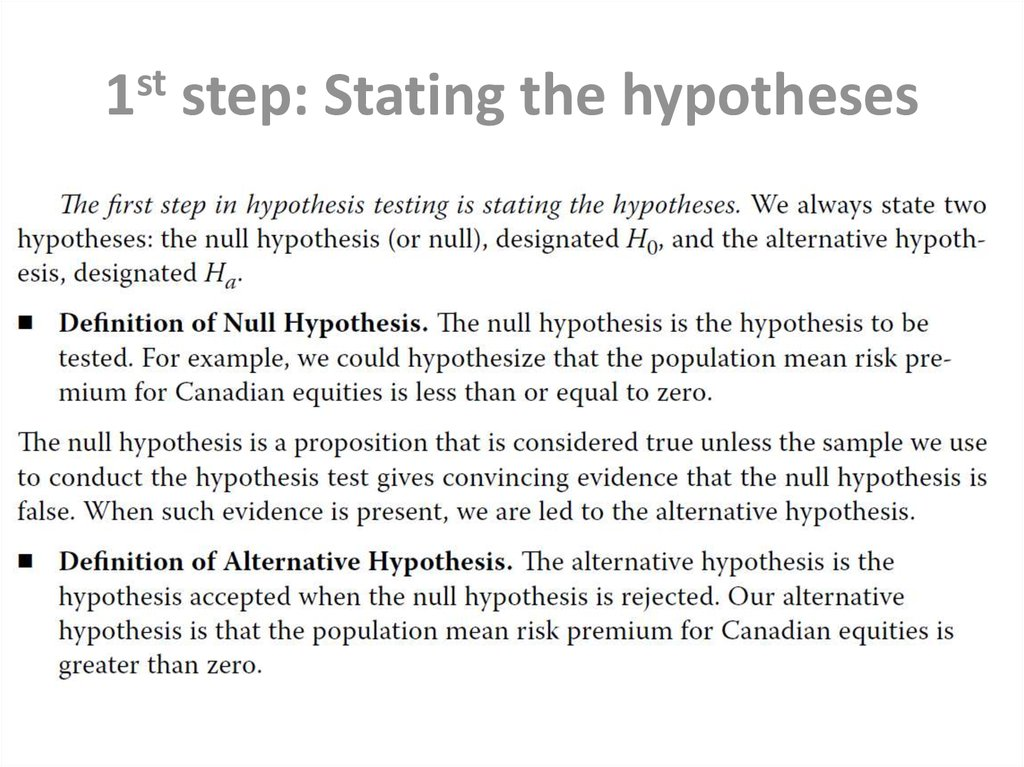# Hypothesis testing

## 1. Hypothesis Testing

By Dias Kulzhanov

## 6. 3rd: Specifying the significance level

• The level of significance reflects how much sample evidence we
require to reject the null. Analogous to its counterpart in a court of
law, the required standard of proof can change according to the
nature of the hypotheses and the seriousness of the consequences of
making a mistake. There are four possible outcomes when we test a
null hypothesis:
• The probability of a Type I error in testing a hypothesis is denoted by
the Greek letter alpha, α. This probability is also known as the level of
significance of the test.

## 7. 4th: Stating the decision rule

• A decision rule consists of determining the rejection points (critical
values) with which to compare the test statistic to decide whether to
reject or not to reject the null hypothesis. When we reject the null
hypothesis, the result is said to be statistically significant.

## 8. 5th: Collecting the data and calculating the test statistic

• Collecting the data by sampling the population
6th: Making the statistical decision
• To reject or not
7th: Making the economic or
investment decision
• The first six steps are the statistical steps. The final decision concerns
our use of the statistical decision.
• The economic or investment decision takes into consideration not
only the statistical decision but also all pertinent economic issues.

## 9. p-Value

• The p-value is the smallest level of significance at which the null
hypothesis can be rejected. The smaller the p-value, the stronger the
evidence against the null hypothesis and in favor of the alternative
hypothesis. The p-value approach to hypothesis testing does not
involve setting a significance level; rather it involves computing a pvalue for the test statistic and allowing the consumer of the research
to interpret its significance.

## 10. Tests Concerning a Single Mean

• For hypothesis tests concerning the population mean of a normally
distributed population with unknown (known) variance, the
theoretically correct test statistic is the t-statistic (z-statistic). In the
unknown variance case, given large samples (generally, samples of 30
or more observations), the z-statistic may be used in place of the tstatistic because of the force of the central limit theorem.
• The t-distribution is a symmetrical distribution defined by a single
parameter: degrees of freedom. Compared to the standard normal
distribution, the t-distribution has fatter tails.

## 14. Tests Concerning Differences between Means

• When we want to test whether the observed difference between
two means is statistically significant, we must first decide whether
the samples are independent or dependent (related). If the samples
are independent, we conduct tests concerning differences between
means. If the samples are dependent, we conduct tests of mean
differences (paired comparisons tests).
• When we conduct a test of the difference between two population
means from normally distributed populations with unknown
variances, if we can assume the variances are equal, we use a t-test
based on pooling the observations of the two samples to estimate
the common (but unknown) variance. This test is based on an
assumption of independent samples.

## 17. Tests Concerning Mean Differences

• In tests concerning two means based on two samples that are not
independent, we often can arrange the data in paired observations
and conduct a test of mean differences (a paired comparisons test).
When the samples are from normally distributed populations with
unknown variances, the appropriate test statistic is a t-statistic. The
denominator of the t-statistic, the standard error of the mean
differences, takes account of correlation between the samples.

## 20. Tests Concerning a Single Variance

• In tests concerning the variance of a single, normally distributed
population, the test statistic is chi-square (χ2) with n − 1 degrees of
freedom, where n is sample size.

## 21.

• Rejection Points for Hypothesis Tests on the
Population Variance.

## 22. Tests Concerning the Equality (Inequality) of Two Variances

• For tests concerning differences between the variances of two
normally distributed populations based on two random, independent
samples, the appropriate test statistic is based on an F-test (the ratio
of the sample variances).

## 25. NONPARAMETRIC INFERENCE

• A parametric test is a hypothesis test concerning a parameter or a
hypothesis test based on specific distributional assumptions. In contrast,
a nonparametric test either is not concerned with a parameter or makes
minimal assumptions about the population from which the sample
comes.
• A nonparametric test is primarily used in three situations: when data do
not meet distributional assumptions, when data are given in ranks, or
when the hypothesis we are addressing does not concern a parameter.

## 26. Tests Concerning Correlation: The Spearman Rank Correlation Coefficient

• The Spearman rank correlation coefficient is essentially equivalent to
the usual correlation coefficient calculated on the ranks of the two
variables (say X and Y) within their respective samples. Thus it is a
number between −1 and +1, where −1 (+1) denotes a perfect inverse
(positive) straight-line relationship between the variables and 0
represents the absence of any straight-line relationship (no
correlation). The calculation of rS requires the following steps: